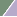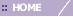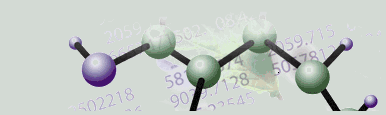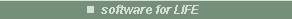Platinum

# Discretization

Overview

Discretization is the process of converting real gene expression data into a typically small number of finite values (e.g. high, medium, low). The variation in the original data is maintained in the discretized dataset. Discretization is a necessary precursor to using association mining algorithms such as SLAM™ to find associations.

Discretization is accomplished by assigning each value in a dataset to a bin. The data ranges (bin boundaries) and number of bins are set on the Discretization parameters dialog.

Quantile Discretization

• In quantile discretization each bin receives an equal number of data values. The data range of each bin varies according to the data values it contains.

Range Discretization

• In range discretization the data range of each bin is equal. The number of data values in each bin varies according to the bin range.

Discretization Target

Discretization can be based on the genes, samples or all of the data in a dataset.

• Per Gene: each gene is divided up into appropriate ranges.

• Per Sample: each sample is divided up into appropriate ranges.

• All Data: all values in the dataset are used to determine the bin ranges.

Actions

1. Click a dataset in the Experiments navigator. The item is highlighted.

2. Click the Discretize Data toolbar icon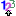, or select Discretize Data from the Predict menu, or right-click the item and select Discretize Data from the shortcut menu. The Discretization dialog is displayed.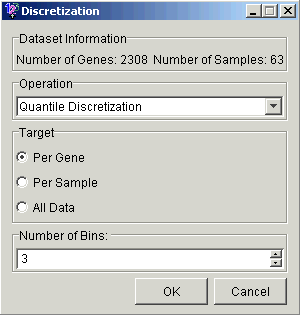3. Set the parameters.

 Parameter Description Operation Type of discretization: Quantile or Range. Target Discretize Per Gene, Per Sample or All Data. Number of Bins The number of discrete groups (bins) to put the values into.

4. Click OK. The Experiment Progress dialog is displayed. It is dynamically updated as the Discretization operation is performed. To cancel the Discretization operation, click the Cancel button.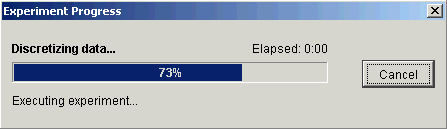Upon successful completion, a new dataset is added under the original dataset in the Experiments navigator.

Related Topics:

ANN Classification and Prediction Overview

SLAM™